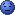# Thread: Parking garage charges [RESOVLED]

1. ##Parking garage charges [RESOVLED]

I got this problem. Here goes: (by the way, check the formula to see if it is right. I'm not 100% sure.

A parking garage charges a \$2.00 minimum fee to park for up to 3 hours. The garage charges an additional \$0.50 for each hour after 3. The maximum charge for any given 24-hour period is \$10.00. Assume that no car parks for more than 24 hours.

Calculate and print the parking charges for each of 3 customers who parked their cars in the garage. Print the grand total of all reciepts. Use a seperate function (cacluateCharges) to determine the charge for each customer.

ex.
Car 1 parks for 1.5 hours. Charge is \$2.00.
Car 2 parks for 4 hours. Charge is \$2.50.
Car 3 parks for 24 hours. Charge is \$10.00

Code:
```#include <iostream.h>

double calculateCharges( void );

int main()
{
double car1, car2, car3;
double car1c, car2c, car3c;

cout << "Please input the amount of hours car 1 was parked: \n";
cin >> car1;
car1c = calculateCharges();
cout << "\nCharge for car1 is: " << car1c << endl;

cout << "Please input the amount of hours car 2 was parked.";
cin >> car2;
car2c = calculateCharges();
cout << "\nCharge for car2 is: " << car2c << endl;

cout << "Please input the amount of hours car 3 was parked.";
cin >> car3;
car3c = calculateCharges();
cout << "\nCharge for car3 is: " << car3c << endl;

return 0;
}

double calculateCharges( void )
{
double parkTime = 3.0, fee = 2.00;

if (parkTime > 3.0)
fee = (fee - 2.00 + parkTime) * .50;

else if (parkTime >= 24.0)
fee = 10.00;

return fee;
}```
I can't figure out whats wrong. When I run it. It says the total is 2.00 for all cars. Maybe I shouldn't decalare fee as \$2.00?Reply With Quote

2. double calculateCharges( void )
{
double parkTime = 3.0, fee = 2.00;

if (parkTime > 3.0)
fee = (fee - 2.00 + parkTime) * .50;

else if (parkTime >= 24.0)
fee = 10.00;

return fee;
}

the line in red is the error, assumably...every call of that function assumes you parked 3 hours...no matter wut

the brown line is never true, cuz of the problem

try this
PHP Code:
``` double calculateCharges(double parkTime ) {     double fee = 2.00;     if (parkTime > 3.0)         fee = (fee - 2.00 + parkTime) * .50;     else if (parkTime >= 24.0)         fee = 10.00;     return fee; }  ```
now pass the park time with each car when calling that function.Reply With Quote

3. hmm~
Code:
```Compiling...
ParkingExcericise.cpp
ParkingExcericise.cpp(13) : warning C4700: local variable 'car1c' used without having been initialized
ParkingExcericise.cpp(18) : warning C4700: local variable 'car2c' used without having been initialized
ParkingExcericise.cpp(23) : warning C4700: local variable 'car3c' used without having been initialized

ParkingExcericise.obj - 0 error(s), 3 warning(s)```
I know it gets annoying if I re-post my code. But I did change a little bit of it. Maybe I changed it the wrong way. I am still learning and just finished the chapter on functions. Which explains why I am doing a newbie program.

Code:
```#include <iostream.h>

double calculateCharges( double );

int main()
{
double grandTotal;
double car1, car2, car3,
car1c, car2c, car3c;

cout << "Please input the amount of hours car 1 was parked: \n";
cin >> car1;
car1c = calculateCharges(car1c);
cout << "\nCharge for car1 is: " << car1c << endl;

cout << "Please input the amount of hours car 2 was parked.";
cin >> car2;
car2c = calculateCharges(car2c);
cout << "\nCharge for car2 is: " << car2c << endl;

cout << "Please input the amount of hours car 3 was parked.";
cin >> car3;
car3c = calculateCharges(car3c);
cout << "\nCharge for car3 is: " << car3c << endl;

grandTotal = car1c + car2c + car3c;
cout << "\n\nGrand total for the day is: " << grandTotal << endl;

return 0;
}

double calculateCharges(double parkTime)
{
double fee = 2.00;

if (parkTime > 3.0)
fee = (fee - 2.00 + parkTime) * .50;

else if (parkTime >= 24.0)
fee = 10.00;

return fee;
}```Reply With Quote

4. PHP Code:
``` //I made a few changes. //Find them yourself, this should work though. #include <iostream.h> double calculateCharges(double parkTime); int main() {     double grandTotal=0;     double car1=0, car2=0, car3=0;                    double car1c=0, car2c=0, car3c=0;          cout << "Please input the amount of hours car 1 was parked: \n";     cin >> car1;     car1c = calculateCharges(car1);     cout << "\nCharge for car1 is: " << car1c << endl;     cout << "Please input the amount of hours car 2 was parked.";     cin >> car2;     car2c = calculateCharges(car2);     cout << "\nCharge for car2 is: " << car2c << endl;     cout << "Please input the amount of hours car 3 was parked.";     cin >> car3;     car3c = calculateCharges(car3);     cout << "\nCharge for car3 is: " << car3c << endl;                 //you don't need to typecast double to double                //well you might for cout below, but try without first               grandTotal = car1c + car2c + car3c;               cout << "\n\nGrand total for the day is: " << grandTotal << endl;     return 0; } double calculateCharges(double parkTime)  {      double fee = 2.00;      if (parkTime > 3.0)          fee = (fee - 2.00 + parkTime) * .50;      else if (parkTime >= 24.0)          fee = 10.00;      return fee;  }  ```Reply With Quote

5. omg. You make it seem so easy! -_- I feel so stupid now. I spent 30 minutes just coming up with the formula.. Thanks a million. Now I go to brush up on arrays.and they were confusing...I should have realized the obvious....initialize them to 0 first. >.<Reply With Quote

6. Also notice you were calling your calculate cost function with the wrong variable. You were calling it with the car1c var, not the car1 var which was the hours.

No problem, enjoy. Glad to see more people learning c++
Have fun, it is a fun language.Reply With Quote

7. The initializing wasn't even necessary here, the problem was the wrong parameter passing.Reply With Quote

8. When you say parameter passing you mean what is in the parenthesis from when I call the function to main()?Reply With Quote

9. The red part is the parameter.
Code:
`car1c = calculateCharges(car1);`Reply With Quote

10. To avoid problem as such I would suggest better variable names like for hours parked maybe

hours_car1
hours_car2
hours_car3

and for the fee for each car
total_car1, fee_car1, cost_car1, or just car1 because nothing else looks the same.

car1c and car1 are to closely related...hard to find errors that way.Reply With Quote

####Posting Permissions

• You may not post new threads
• You may not post replies
• You may not post attachments
• You may not edit your posts
•